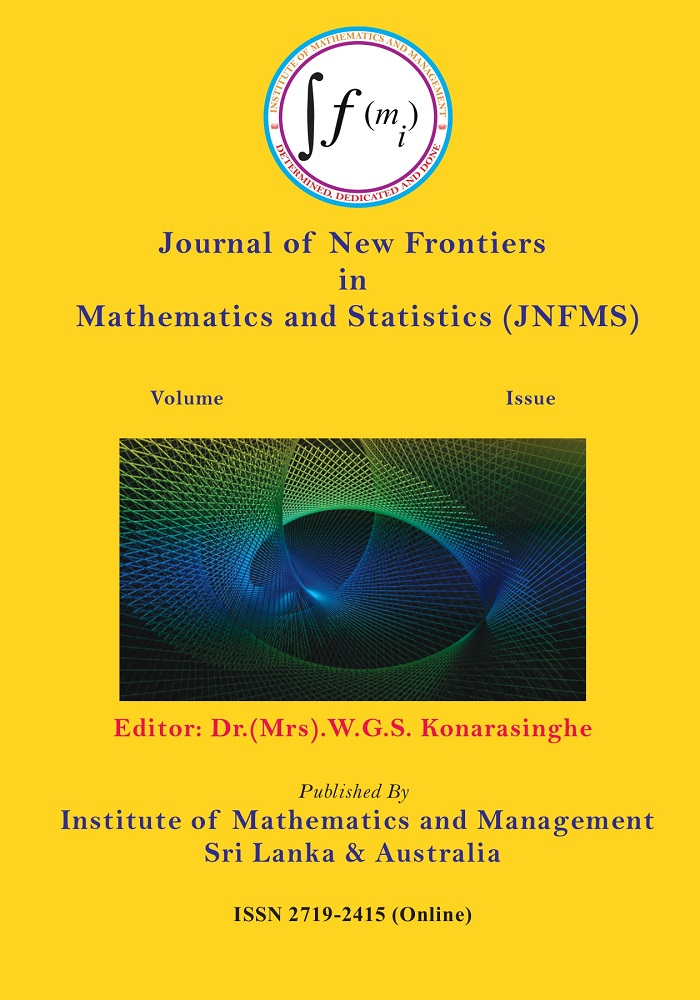# Journal of New Frontiers in Mathematics and Statistics ( JNFMS)Impact Factor: 1.67 (2022)

Aim and Scope of the Journal
Journal of New Frontiers in Mathematics and Statistics (JNFMS) is an international peer-reviewed academic journal facilitating communications among Mathematicians and Statisticians with cross-disciplinary research interests. JNFMS provides an outlet for the increasing flow of interdisciplinary research across mathematics and statistics. It is an essential material for mathematicians, statisticians, academicians, graduate students, and scientists in science and technology policy, research, and development management in pure and applied mathematics and statistics research.
Mathematics
Pure Mathematics, Applied Mathematics, Mathematical Modeling, Mathematics Education, Mathematical Physics, Mathematics for Business & Economics, Mathematics for Finance, Actuarial Mathematics, Industrial Mathematics, Mathematical Physics, Mathematical Chemistry, Mathematical Medicine and Biology, Engineering Mathematics, Computer Mathematics, Mathematics for Social Sciences, Operations Research, Algebra & Logic, Functional Analysis, Set Theory, Matrix Algebra, Number Theory, GroupTheory, MeasureTheory, Geometry, Cryptography, Numerical Analysis, Fluid Mechanics, Heat and Mass Transfer, Differential Equations, Optimization, Topology, Game Theory, etc. and allied subjects.
Statistics
Statistical Theory, Applied Statistics, Bayesian Statistics, Bio-Statistics, Medical Statistics, Social Statistics, Business Statistics, Design and Analysis of Experiments, Stochastic Process, Time Series Analysis, Multivariate Statistical Analysis, Decision Theory, etc Allied Subjects.
Call for Papers
Original research papers and review papers in Mathematics, Statistics, and related disciplines from Undergraduate and Post Graduate Students, Research Scholars, Faculty, Scientists, etc.,  are invited for presentation and publication.
Publication Frequency
The publication frequency of JNFMS is Bi-annual.
Referencing
APA Reference Style

View Articles ISSN 2719 -2415 (Online)
Vol.3(2023), Issue 1
Sama Radial Indicator (SRI) for Measuring Qualitative Variables.Journal of New Frontiers in Mathematics and Statistics, Vol.3(1),1-10.
W.G.S.Konarasinghe
Vol.2(2021), Issue 2
M.G.Ogungbenle
Model Development for Damped and Forced Type of Oscillations in Time Series.Journal of New Frontiers in Mathematics and Statistics, Vol.2(2),20-35
W.G.S.Konarasinghe,K.M.U.B.Konarasinghe
Vol.2(2021), Issue 1
Action of a Semiring to Encrypt Asymmetric Key – Elgamal.Journal of New Frontiers in Mathematics and Statistics, Vol.2(1),1-10.
S.Nivetha, M.Chandramouleeswaran
Chromatic Polynomials of S-Valued Graphs.Journal of New Frontiers in Mathematics and Statistics, Vol.2(1),11-20.
T.V.G.Shriprakash, M.Chandramouleeswaran
Vol.1(2020), Issue 1
Comparison of Forecasting ability of Sama Circular Model, ARIMA, and Decomposition Techniques.Journal of New Frontiers in Mathematics and Statistics, Vol.1(1),1-15.
W.G.S.Konarasinghe
Identifying Factors Associated with Price Categories of Motorcycles in Sri Lanka using Discriminant Analysis. Journal of New Frontiers in Mathematics and Statistics, Vol.1(1),16-25.
P.A.L. Chanika, A.M.C.H. Attanayake, M.D.N. Gunaratne